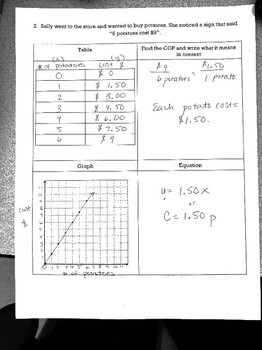Subject
Resource Type
File Type

PDF

(1 MB)
Product Rating
Standards
• Product Description
• StandardsNEW

This link sheet has students read a real world situation, create a table of values, graph the ordered pairs, and then write the equation using the constant of proportionality. This was a great way to introduce the equation of direct variation and using y=Kx. This a 2 page document and has the answer key with it!

Represent proportional relationships by equations. For example, if total cost 𝘵 is proportional to the number 𝘯 of items purchased at a constant price 𝘱, the relationship between the total cost and the number of items can be expressed as 𝘵 = 𝘱𝘯.
Identify the constant of proportionality (unit rate) in tables, graphs, equations, diagrams, and verbal descriptions of proportional relationships.
Recognize and represent proportional relationships between quantities.
Solve unit rate problems including those involving unit pricing and constant speed. For example, if it took 7 hours to mow 4 lawns, then at that rate, how many lawns could be mowed in 35 hours? At what rate were lawns being mowed?
Make tables of equivalent ratios relating quantities with whole-number measurements, find missing values in the tables, and plot the pairs of values on the coordinate plane. Use tables to compare ratios.
Total Pages
N/A
N/A
Teaching Duration
30 minutes
Report this Resource to TpT
Reported resources will be reviewed by our team. Report this resource to let us know if this resource violates TpT’s content guidelines.